# An Introduction to Ridge, Lasso, and Elastic Net Regression

### A guide to Ridge, Lasso, and Elastic Net Regression and applying it in R

Regression analysis is a statistical technique that models and approximates the relationship between a dependent and one or more independent variables. This article will quickly introduce three commonly used regression models using R and the Boston housing data-set: Ridge, Lasso, and Elastic Net.

First we need to understand the basics of regression and what parameters of the equation are changed when using a specific model. Simple linear regression, also known as ordinary least squares (OLS) attempts to minimize the sum of error squared. The error in this case is the difference between the actual data point and its predicted value.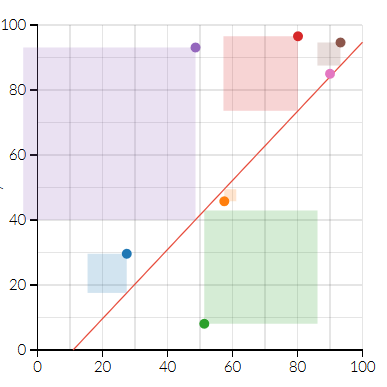Visualization of the squared error (from Setosa.io)

The equation for this model is referred to as the cost function and is a way to find the optimal error by minimizing and measuring it. The gradient descent algorithm[link] is used to find the optimal cost function by going over a number of iterations. But the data we need to define and analyze is not always so easy to characterize with the base OLS model.Equation for least ordinary squares

One situation is the data showing multi-collinearity, this is when predictor variables are correlated to each other and to the response variable. To picture this let’s say we’re doing a study that looks at a response variable — patient weight, and our predictor variables would be height, sex, and diet. The problem here is that height and sex are also correlated and can inflate the standard error of their coefficients which may make them seem statistically insignificant.

To produce a more accurate model of complex data we can add a penalty term to the OLS equation. A penalty adds a bias towards certain values. These are known as L1 regularization(Lasso regression) and L2 regularization(ridge regression).

The best model we can hope to come up with minimizes both the bias and the variance: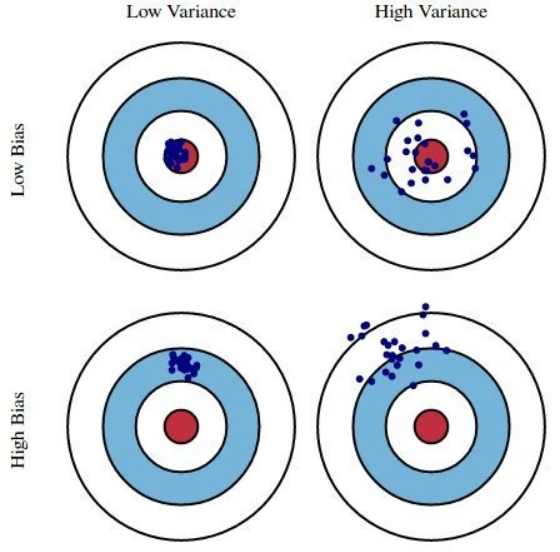Variance/bias trade off (KDnuggets.com)

### Ridge Regression

Ridge regression uses L2 regularization which adds the following penalty term to the OLS equation.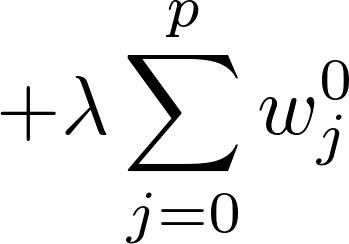L2 regularization penalty term

The L2 term is equal to the square of the magnitude of the coefficients. In this case if lambda(λ) is zero then the equation is the basic OLS but if it is greater than zero then we add a constraint to the coefficients. This constraint results in minimized coefficients (aka shrinkage) that trend towards zero the larger the value of lambda. Shrinking the coefficients leads to a lower variance and in turn a lower error value. Therefore Ridge regression decreases the complexity of a model but does not reduce the number of variables, it rather just shrinks their effect.

### Lasso regression

Lasso regression uses the L1 penalty term and stands for Least Absolute Shrinkage and Selection Operator. The penalty applied for L2 is equal to the absolute value of the magnitude of the coefficients: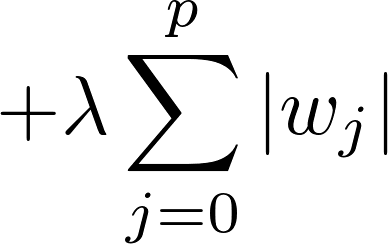L1 regularization penalty term

Similar to ridge regression, a lambda value of zero spits out the basic OLS equation, however given a suitable lambda value lasso regression can drive some coefficients to zero. The larger the value of lambda the more features are shrunk to zero. This can eliminate some features entirely and give us a subset of predictors that helps mitigate multi-collinearity and model complexity. Predictors not shrunk towards zero signify that they are important and thus L1 regularization allows for feature selection (sparse selection).

### Elastic Net

A third commonly used model of regression is the Elastic Net which incorporates penalties from both L1 and L2 regularization:Elastic net regularization

In addition to setting and choosing a lambda value elastic net also allows us to tune the alpha parameter where 𝞪 = 0 corresponds to ridge and 𝞪 = 1 to lasso. Simply put, if you plug in 0 for alpha, the penalty function reduces to the L1 (ridge) term and if we set alpha to 1 we get the L2 (lasso) term. Therefore we can choose an alpha value between 0 and 1 to optimize the elastic net. Effectively this will shrink some coefficients and set some to 0 for sparse selection.

### Preparing the data

We will be using the following packages:

`library(tidyverse)library(caret)library(glmnet)`

We’ll also be using R’s built in Boston housing market data set as it has many predictor variables

`data(“Boston”, package = “MASS”)`
`#set a seed so you can reproduce the resultsset.seed(1212)`
`#split the data into training and test datasample_size <- floor(0.75 * nrow(Boston))`
`training_index <- sample(seq_len(nrow(Boston)), size = sample_size)`
`train <- Boston[training_index, ]`
`test <- Boston[-training_index, ]`

We also should create two objects to store predictor (x) and response variables (y, median value)

`# Predictorx <- model.matrix(medv~., train)[,-1]`
`# Responsey <- train\$medv`

### Performing Ridge regression

As we mentioned in the previous sections, lambda values have a large effect on coefficients so now we will compute and chose a suitable one.

Here we perform a cross validation and take a peek at the lambda value corresponding to the lowest prediction error before fitting the data to the model and viewing the coefficients.

`cv.r <- cv.glmnet(x, y, alpha = 0)`
`cv.r\$lambda.min`
`model.ridge <- glmnet(x, y, alpha = 0, lambda = cv.r\$lambda.min)`
`coef(model.ridge)`

We can see here that certain coefficients have been pushed towards zero and minimized while RM (number of rooms) has a significantly higher weight than the rest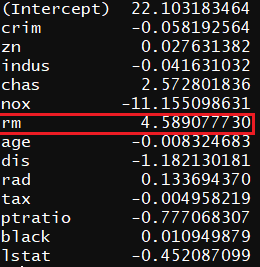Ridge regression coefficients

We now look at how our model performs by using our test data on it.

`x.test.ridge <- model.matrix(medv ~., test)[,-1]`
`predictions.ridge <- model.ridge%>% predict(x.test.ridge)%>% as.vector()`
`data.frame(  RMSE.r = RMSE(predictions.ridge, test\$medv),  Rsquare.r = R2(predictions.ridge, test\$medv))`

### Performing Lasso regression

The steps will be identical to what we have done for ridge regression. The value of alpha is the only change here (remember 𝞪 = 1 denotes lasso)

`cv.l <- cv.glmnet(x, y, alpha = 1)`
`cv.l\$lambda.min`
`model.lasso <- glmnet(x, y, alpha = 1, lambda = cv.l\$lambda.min)coef(model.lasso)`
`x.test.lasso <- model.matrix(medv ~., test)[,-1]predictions.lasso <- model.lasso %>%predict(x.test.lasso) %>% as.vector()`
`data.frame(RMSE.l = RMSE(predictions.lasso, test\$medv),Rsquare.l = R2(predictions.lasso, test\$medv))`

### Performing Elastic Net regression

Performing Elastic Net requires us to tune parameters to identify the best alpha and lambda values and for this we need to use the caret package. We will tune the model by iterating over a number of alpha and lambda pairs and we can see which pair has the lowest associated error.

`model.net <- train(    medv ~., data = train, method = "glmnet",    trControl = trainControl("cv", number = 10),    tuneLength = 10)`
`model.net\$bestTunecoef(model.net\$finalModel, model.net\$bestTune\$lambda)`
`x.test.net <- model.matrix(medv ~., test)[,-1]`
`predictions.net <- model.net %>% predict(x.test.net)`
`data.frame(RMSE.net = RMSE(predictions.net, test\$medv),Rsquare.net = R2(predictions.net, test\$medv))`

### Conclusion

We can see that the R mean-squared values using all three models were very close to each other, but both did marginally perform better than ridge regression (Lasso having done best). Lasso regression also showed the highest R² value.An Introduction to Ridge, Lasso, and Elastic Net Regression was originally published in Hacker Noon on Medium, where people are continuing the conversation by highlighting and responding to this story.

Read about withdrawable no deposit bonus and make profit trading now